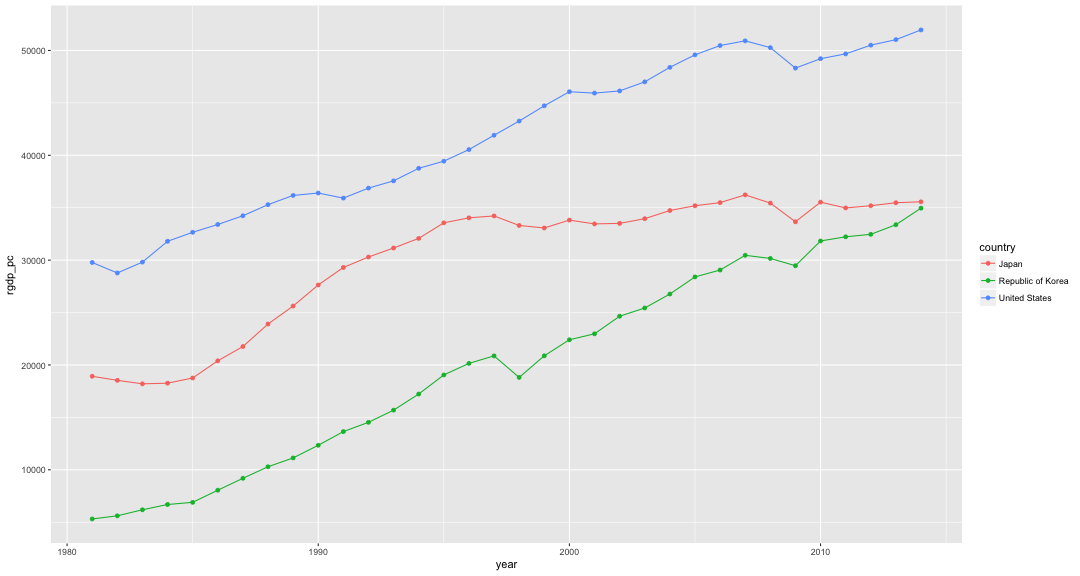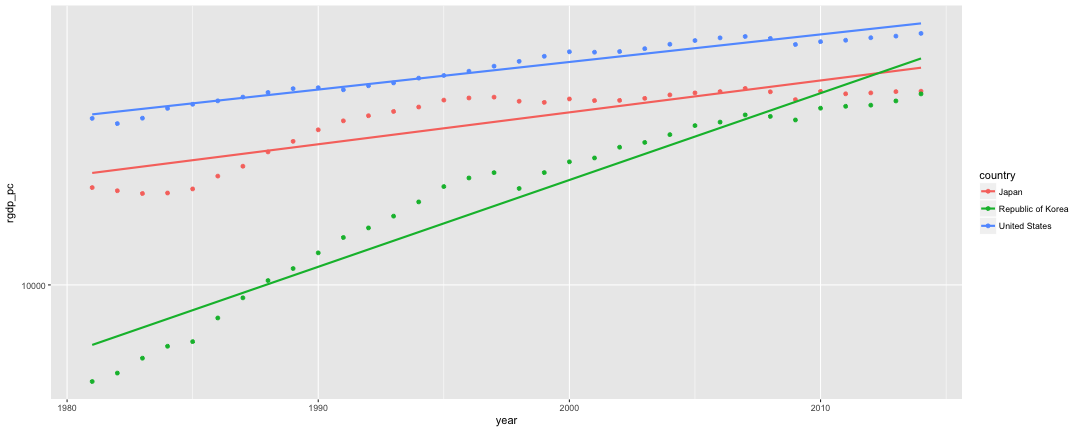# Macroeconomics Q4

Kenji Sato
2016-12-07

### Welcome to Macroeconomics (Q4)!

• Name:
• Kenji Sato
• PLEASE DO NOT call me “Professor!”
• “Kenji” or “Sato-san” are preferable. “Sato-sensei” is acceptable.
• Fields of Interest:
• Macroeconomic Dynamics
• Economics of Innovation
• Comparative Statics/Dynamics
• For course mechanics, take a look at the handout.

### Welcome to Macroeconomics (Q4)!

• Office hours
• Textbook
• Schedule
• Course websites

### Welcome to Macroeconomics (Q4)!

• Macroeconomics is a study of such aggregate economic evariables as …
• Gross Domestic Product (GDP)
• Growth Rate
• Unemployment Rate
• Inflation Rate
• Income Inequality

### Welcome to Macroeconomics (Q4)!

• In this course, you are going to study …
• basic machinery of graduate-level macroeconomics;
• how to code computer programs to compare theory with data;
• theories on how GDP and growth rates move.
• To learn how to solve growth models, you will need to learn
• differential equations and difference equations, and
• dynamic optimization.

### Limit

• Because of the limited time, this course will focus on a study of economic growth theory.
• The foundation of modern macroeconomic research.
• If we have time, we will move on to the study of innovation or business cycles.
• No money, unemployment or inequality.

### Call for Enrollment

• You are highly recommended to take more advanced/applied courses together with this course.
• Inequality and Macroeconomics by Professor Dr. Reto Föllmi, from January 26 to 31.
• Please go to Kyoumu for enrollment!

### Programing Languages

After taking Watkins's courses, I believe you are familiar with R programing language.

• I will also use R when I show you data.
• Highly recommended to type yourself to reproduce plots.
• Building muscle memory is a necessary part of climbing up the learning curve.
• Sometimes I will use Python for performing simulations.
• Because I like Python.

### Penn World Table, Ver. 8

• Feenstra, Inklaar and Timmer (2015) “The next generation of the Penn World Table,” American Economic Review 105(10). 3150–82.
library(readstata13)
library(dplyr)
library(ggplot2)


### Penn World Table, Ver. 8 (cont'd)

You can easily analyse the data with R + dplyr.

pwt8 %>%
filter(country == "Japan", year > 2010) %>%
mutate(rgdp_per_capita = rgdpo / pop) %>%
select(year, rgdp_per_capita)

  year rgdp_per_capita
1 2011        34979.34
2 2012        35191.24
3 2013        35476.46
4 2014        35566.22


Unit: 2011US\$

Note: With R it is difficult to see the descriptive labels that STATA variables have. Install gretl to work around.

### Real GDP per capita: Data processing

We are interested in evolution of real GDP per person (per capita). Becase it is a nice statistic to measure the standard of living for a country.

Let's compare those of three countries since 1980.

rgdp <- pwt8 %>%
filter(countrycode %in% c("JPN","USA", "KOR"),
year > 1980) %>%
mutate(rgdp_pc = rgdpo / pop) %>%
select(country, year, rgdp_pc) %>%
as.data.frame


### Real GDP per capita: Plotting

ggplot(rgdp, aes(x=year, y=rgdp_pc, color=country)) +
geom_point() + geom_line(aes(group=country))### GDP Growth

When studying the growth rates, semi-log scale is helpful.

rgdp %>% ggplot(aes(x=year, y=rgdp_pc, color=country)) + geom_point() +
scale_y_log10() +  # Plot in semi-log scale
geom_smooth(method = "lm", se = FALSE)### Why log-scale?

\begin{aligned} & \text{Gross annual growth rate from 2000 to 2010} \\ &= \left(\frac{GDP_{2010}}{GDP_{2000}}\right)^{1/10} \end{aligned}

And so, ….

\begin{aligned} & \log (\text{Gross annual growth rate from 2000 to 2010}) \\ &= \frac{\log GDP_{2010} - \log GDP_{2000}}{2010 - 2000} \end{aligned}

The slopes of the lines you observed in the semi-log plot correspond to the growth rates of the countries.

### Change in growth rates.

The growth rates change over time.

rgdp %>% ggplot(aes(x=year, y=rgdp_pc, color=country)) + geom_point() +
scale_y_log10() +  # Plot in semi-log scale
geom_smooth(method="lm", se=FALSE) +
geom_smooth(data=filter(rgdp, year > 1995),
method="lm", se=FALSE)